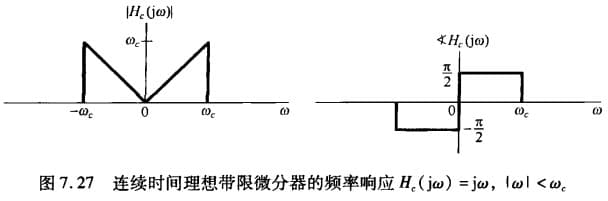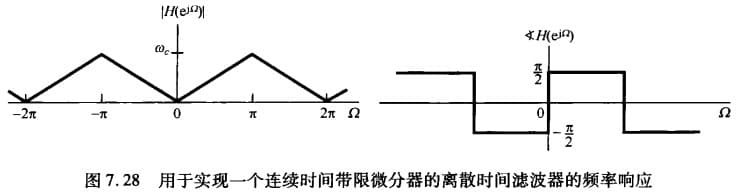# 模数转换与数模转换

$\newcommand{\dif}{\mathop{}\!\mathrm{d}} \newcommand{\xleftrightarrow}{\stackrel{#1}{\longleftrightarrow}} \newcommand{\F}{\mathcal{F}} \newcommand{\ft}{\xleftrightarrow{\F}}$

C/D转换又称为模数转换（A/D），D/C转换又称为数模转换（D/A）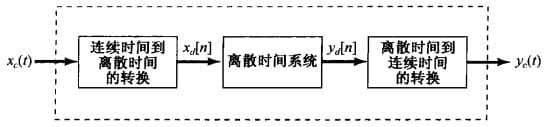• $x_c(t)$ 表示输入的连续信号
• $x_d[n]$ 表示连续信号经过 C/D 转换得到的离散信号
• $y_d[n]$ 表示输出的离散信号
• $y_c(t)$ 表示离散信号经过 D/C 转换得到的连续信号

# C/D转换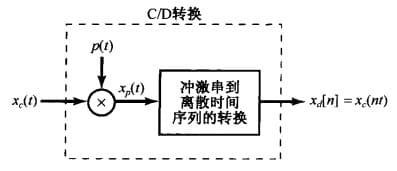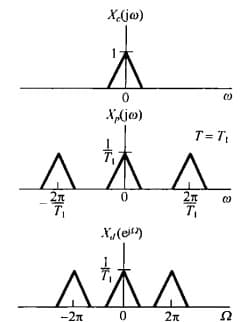$x_p(t)$ 时间轴上原本是以 $T$ 为间隔的，经过这样的变换后，$x_d[n]$ 则是变为了以 $1$ 为间隔。我们将这种变换称为 归一化

# D/C转换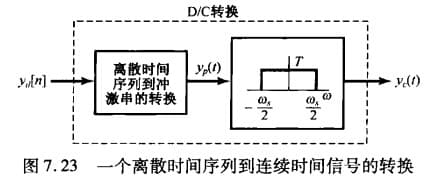# 利用离散系统处理连续信号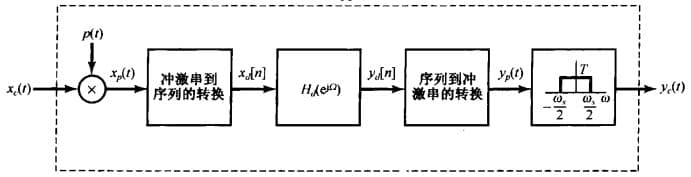C/D转化 与 D/C转换互为逆过程，通过这个我们就能用离散系统处理连续信号，也就是说，上图虚线框中的系统相当于一个线性时不变系统。在这里提出一个问题：$x_c(t)p(t)$ 并不是一个时不变环节，为什么整个系统还能等效为一个线性时不变系统？

## 数字微分器